Next: Sample session with cdd+ Up: Voronoi Diagram and Delaunay Previous: Sample session with cdd+   Contents

## Is it possible to compute only the adjacencies of Voronoi cells in the Voronoi diagram efficiently?

Yes, it can be done very efficiently by linear programming (LP), and very importantly this can be done for very large scale problems, with practically no bounds on the size with an efficient LP solver.

The method is simple. The lifting technique we described in 3.2 immediately gives the idea. Recall that the Voronoi diagram of a set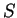of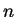points in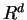is the projection of the following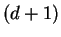-polyhedron tospace of the first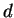components.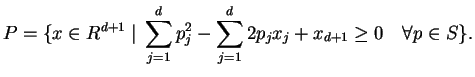For simplicity, denote it as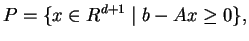where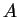is a given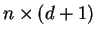matrix and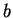is a-vector. Now for each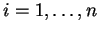, consider the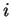th facet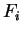of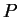: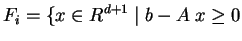and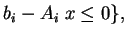(7)

Two facetsand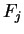are called adjacent if the intersection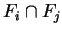is a facet of both, i.e. has dimension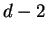. An equivalent definition is: they are adjacent if (*) the facetbecomes larger once the facetis removed from the polyhedron, i.e. the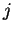th inequality is removed from the system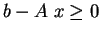.

It is easy to see that two Voronoi cellsand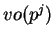are adjacent if and only if the corresponding facetsandare adjacent in the polyhedron. Now, we formulate the following LP for any distinct,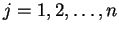:(8)

where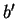is equal toexcept forth component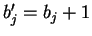. The new inequality system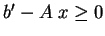is simply a modification of the original system obtained by relaxing theth inequality a little bit. An important remark is, by definition (*),andare adjacent if and only if the objective value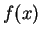is negative at an optimum solution. Thus we formulated the Voronoi adjacency computation as an LP problem.

How much do we gain by using LP for the adjacency computation, instead of computing the whole Voronoi diagram? A lot. It is hard to exaggerate this, because the LP (8) (in fact any LP) is solvable in polynomial time, whereas the associated Voronoi computation is exponential inand. Using the standard simplex method, the time complexity of solving an LP is not polynomial, but the practical complexity is roughly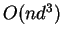.

SubsectionsNext: Sample session with cdd+ Up: Voronoi Diagram and Delaunay Previous: Sample session with cdd+   Contents
Komei Fukuda 2004-08-26